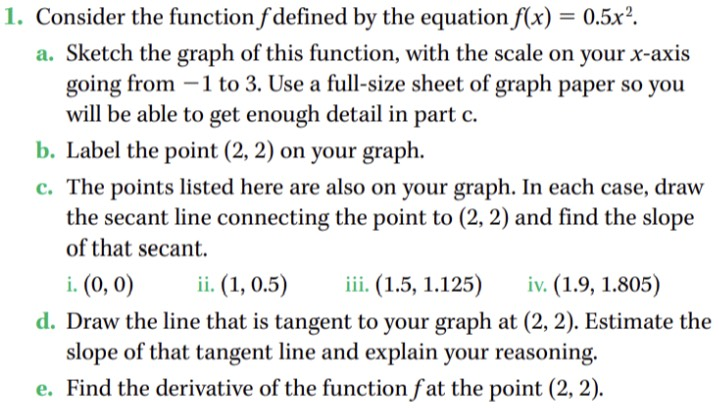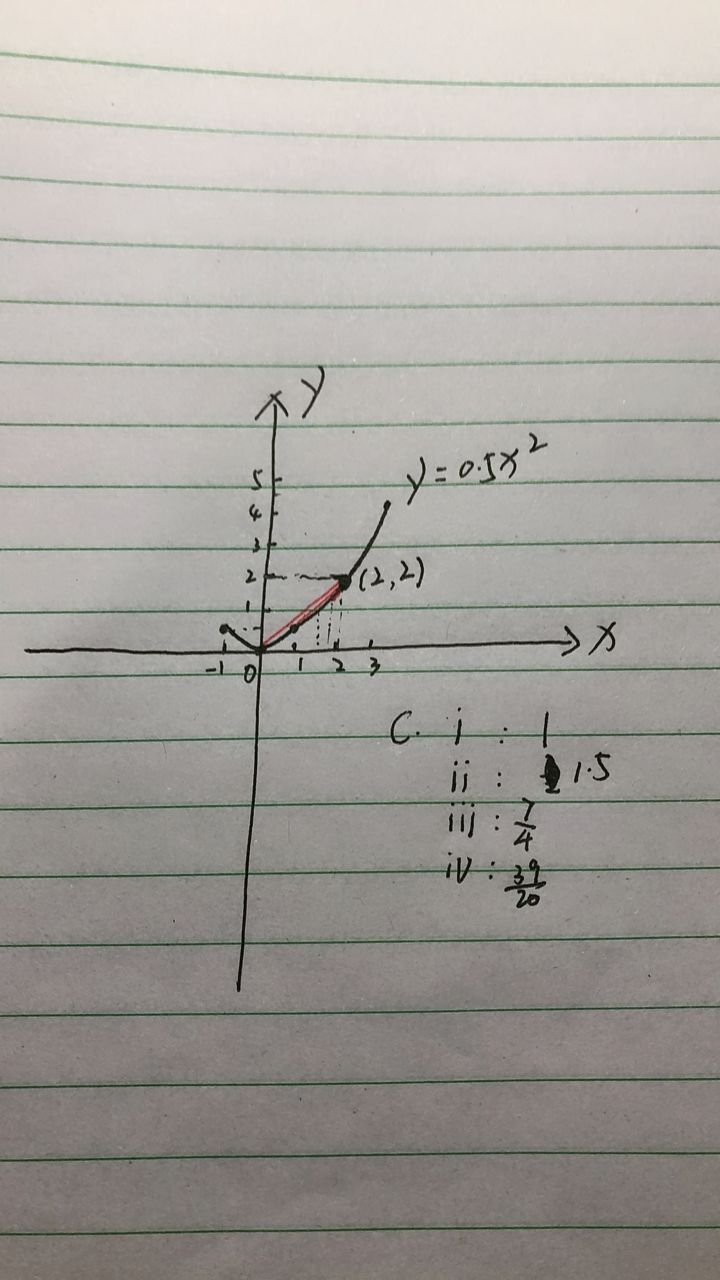### ¿Todavía tienes preguntas de matemáticas?

Pregunte a nuestros tutores expertos
Algebra
Pregunta1. Consider the function $$f$$ defined by the equation $$f ( x ) = 0.5 x ^ { 2 }$$ . a. Sketch the graph of this function, with the scale on your $$x$$ -axis going from $$- 1$$ to $$3$$ . Use a full-size sheet of graph paper so you will be able to get enough detail in part c. b. Label the point $$( 2,2 )$$ on your graph. c. The points listed here are also on your graph. In each case, draw the secant line connecting the point to $$( 2,2 )$$ and find the slope of that secant. i. $$( 0,0 )$$ ii. $$( 1,0.5 )$$ iii. $$( 1.5,1.125 )$$ iv. $$( 1.9,1.805 )$$

d. Draw the line that is tangent to your graph at $$( 2,2 )$$ . Estimate the slope of that tangent line and explain your reasoning. e. Find the derivative of the function $$f$$ at the point $$( 2,2 )$$